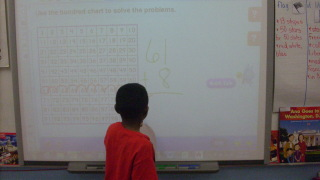# Using a Hundreds Chart to Add

17 teachers like this lesson
Print Lesson

## Objective

SWBAT use a hundred chart to find sums.

#### Big Idea

Hundreds charts allow for children to visually follow along and see how the numbers grow as they apply the counting on strategy to greater numbers.

## Activating Strategy

10 minutes

I begin this lesson using this video/song:

I then write a two digit number on the board.  Discuss which number is in the tens place, and the ones place.  I then give each child a white board and marker (or a piece of paper will work too!).  I challenge them to write other two digit numbers using the following directions:

• Write a two digit number with 0 ones.
• Write a two digit number with the same number of ones and tens.
• Write a two digit number with 6 tens.
• Write a two digit number with 6 ones.

This activity gets them thinking about place value and the importance of have the correct digit in the correct place.

The standard NBT.C.4 includes multiple strategies for adding a two digit number to 100. A hundred chart is another tool that can be used to show children "why addition works." Children are familiar with a counting on strategy for basic facts. Hundred charts allow children to visually follow along and see how the numbers grow as they apply the counting on strategy to greater numbers. They can touch each number as they count on across the row to add ones, or count on down the columns to add tens.

In first grade students need to have multiple opportunities to add and subtract ten and multiple of ten.  When they are exposed to varying strategies they are able to gain a better understanding of place value and are able to look for repeated reasoning (M.P8)

## Teaching Strategies

15 minutes

To start the lesson, I display a hundreds chart and allow students a few minutes to look it over.  As a group we use the chart to count to 100.  We discuss how the numbers appear and talk about any patterns that they observe.  For example:

• How can you use a hundred chart to add ones? (I can move to the right in a row.  Each space is adding 1.)
• How can you use a hundred chart to add tens? (I can move down a column.  Each column is adding 10.)

I read the following problem aloud, or write on board/chart paper:

Alice picks 12 flowers.  Then she picks 4 more.  How many flowers does Alice pick?

I then tell children they can use the hundred chart to solve the problem.  I model by starting at 12 and counting on four spaces to the right.  Ask the students:

• Where did I stop? (16)
• How does using a hundred chart help you count by ones? (As I move to the right across the row, each space shows the number that is one more.)

I then read the next problem:  Jim picks 10 strawberries.  The he picks 20 more strawberries.  How many strawberries does Jim pick?

I like to model how to tens by using the chart – start at 10, and move down 2 columns (counting by tens as you move).  Ask the students:

• Where did I stop (30)
• How does using a hundred chart help you count by tens? (As I move down the column, each space shows the number that is ten more.

I then work through the next problem with children to solve 24 + 4. Have children write the sum.

• If you move across from 24 to 28 on the hundred chart, how many have you added to 24? (4 ones, or 4)

I also work through the next problem with children to solve 31 + 40. Have children write the sum.

• If you move down from 31 to 71 on the hundred chart, how many have you added to 31? (4 tens, or 40)

Then I work through the final problem with children to solve 61 + 8. Have children write the sum.

• If you move across from 61 to 69 on the hundred chart, how many have you added to 61? (8 ones, or 8)

This pictures shows a student demonstrating how to move across the hundred chart to add.## Independent Practice

30 minutes

For the independent activity, I use Use a Hundred Chart to Add_worksheet to check for understanding.

For those struggling students, I put them in a small group and work on more strategies.  Using a hundreds chart we work on more problems together.  For example:

Point to 51 on the hundreds chart.  Model how to add 51 + 4.  The important part of this lesson is to make sure that the students start at 51, and don’t start counting on to add until 52.  (Make sure that they move one space to the right for each number they are adding.)  To get them to understand I have each student put their finger on 51 and we start counting forward together.  We work through more problems together, and adding 10 to a number until they are ready for independent practice.

## Closing/Summarizing

5 minutes

To close out the lesson, I have students write and solve the following in their math journals:

Write a number sentence to add 6 ones to 21.

Write a number sentence to add 6 tens to 21.